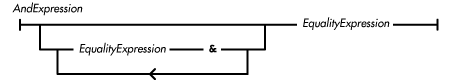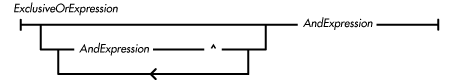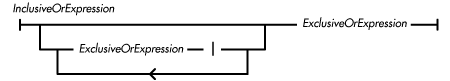start page | rating of books | rating of authors | reviews | copyrights

### 4.10 Bitwise/Logical Operators

The bitwise/logical operators in Java are used for bitwise and logical AND (&), bitwise and logical exclusive OR (^), and bitwise and logical inclusive OR (|) operations. These operators have different precedence; the & operator has the highest precedence of the group and the | operator has the lowest. All of the operators are evaluated from left to right.

The unary operator ~ provides a bitwise negation operation.

### Bitwise/Logical AND Operator &

The bitwise/logical AND operator & produces a pure value that is the AND of its operands. The & operator may appear in a bitwise or logical AND expression:The bitwise/logical AND operator is evaluated from left to right. The operator never throws an exception.

Here is a code example that shows the use of the bitwise AND operator:

```boolean isOdd(int x) {
return (x & 1) == 1;
}
```

The operands of the bitwise/logical AND operator must both be of either an integer type or the type boolean, or a compile-time error occurs.

If both operands are of integer types, the operator performs a bitwise AND operation. The operator may perform type conversions on the operands:

• If either operand is of type long, the other operand is converted to long and the operation produces a long value.

• Otherwise, both operands are converted to int and the operation produces an int value.

The bitwise AND operator produces a pure value that is the bitwise AND of its operands. If the corresponding bits in both of the converted operands are 1s, the corresponding bit in the result is a 1; otherwise the corresponding bit in the result is a 0.

If both operands are of type boolean, the operator performs a logical AND operation. The logical AND operation produces a pure value of type boolean. If both operands are true, the operation produces true; otherwise the operation produces false. This operator differs from the conditional AND operator (&&) because it always evaluates both of its operands, even if its left operand evaluates to false.

### Bitwise/Logical Exclusive OR Operator ^

The bitwise/logical exclusive OR operator ^ produces a pure value that is the exclusive OR of its operands. The ^ operator may appear in a bitwise or logical exclusive OR expression:The bitwise/logical exclusive OR operator is evaluated from left to right. The operator never throws an exception.

The operands of the bitwise/logical exclusive OR operator must both be of either an integer type or the type boolean, or a compile-time error occurs.

If both operands are of integer types, the operator performs a bitwise exclusive OR operation. The operator may perform type conversions on the operands:

• If either operand is of type long, the other operand is converted to long and the operation produces a long value.

• Otherwise, both operands are converted to int and the operation produces an int value.

The bitwise exclusive OR operator produces a pure value that is the bitwise exclusive OR of its operands. If the corresponding bits in the converted operands are both 0 or both 1, the corresponding bit in the result is a 0; otherwise the corresponding bit in the result is a 1.

If both operands are of type boolean, the operator performs a logical exclusive OR operation. The logical exclusive OR operation produces a pure value of type boolean. If either, but not both, operands are true, the operation produces true; otherwise the operation produces false.

### Bitwise/Logical Inclusive OR Operator |

The bitwise/logical inclusive OR operator | produces a pure value that is the inclusive OR of its operands. The | operator may appear in a bitwise or logical inclusive OR expression:The bitwise/logical inclusive OR operator is evaluated from left to right. The operator never throws an exception.

Here is a code example that shows the use of the bitwise inclusive OR operator:

```setFont("Helvetica", Font.BOLD | Font.ITALIC, 18);
```

The operands of the bitwise/logical inclusive OR operator must both be of either an integer type or the type boolean, or a compile-time error occurs.

If both operands are of integer types, the operator performs a bitwise inclusive OR operation. The operator may perform type conversions on the operands:

• If either operand is of type long, the other operand is converted to long and the operation produces a long value.

• Otherwise, both operands are converted to int and the operation produces an int value.

The bitwise inclusive OR operator produces a pure value that is the bitwise inclusive OR of its operands. If the corresponding bits in either or both of the converted operands are 1s, the corresponding bit in the result is a 1; otherwise the corresponding bit in the result is a 0.

If both operands are of type boolean, the operator performs a logical inclusive OR operation. The logical inclusive OR operation produces a pure value of type boolean. If either or both operands are true, the operation produces true; otherwise the operation produces false. This operator differs from the conditional OR operator (||) because it always evaluates both of its operands, even if its left operand evaluates to true.Equality Comparison OperatorsBoolean Operators